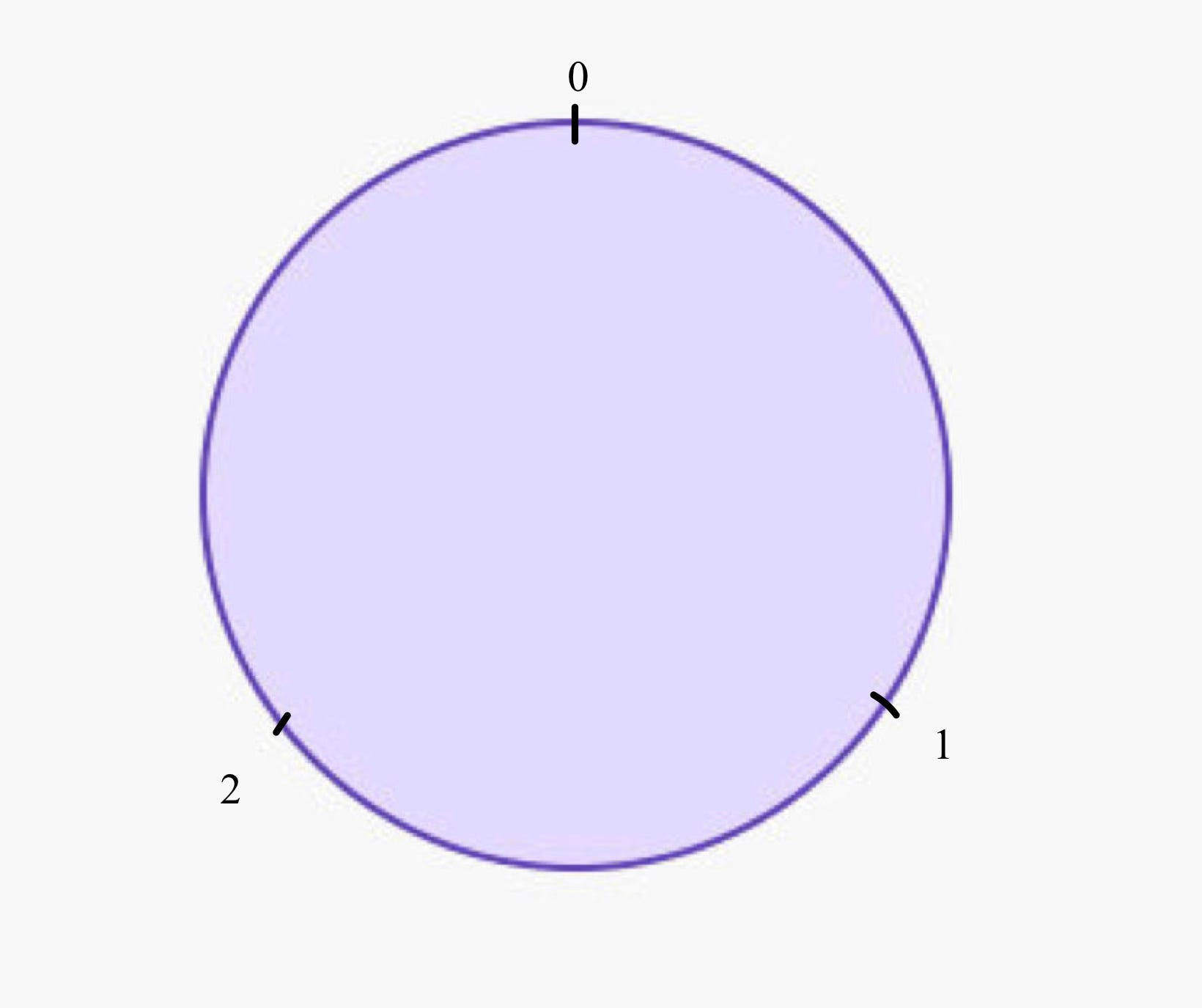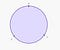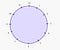# Algebra Demystified: Part two. A closer look at the clock.

“Curiouser and Curiouser” cried Alice.

Mathematics is something we get exposed to from a very early age. The numbers form a fundamental part of understanding the world around us and making sense of it. If numbers did not exist how would we answer basic questions like how old we are, what time is it, and more important existential problems like how much we save on single candy bar when buying a multipack, how many grams of flour we need to make a banana cake?

Many kids learn how to count before they learn the alphabet. When I was little my grandparents taught me how to add and subtract numbers up to ten before I was able to spell my own name. The point is, numbers and counting are an integral part of who we are and what our place in the world is. Numbers have helped us understand so much about our planet and have also helped us establish a sense of order in our lives. However, being the curious species we are, we humans have taken the study of numbers quite far. Since Ancient Greek times different philosophers and scientists have tried to lift the mystery veil surrounding arithmetic and have really tried to understand what properties numbers actually have. Euclid’s “Elements” is the first written resource we are aware of which gives the definition of a prime number. (A little aside — a prime number is a number only divisible by one and itself. These numbers are the building blocks of number theory.)

Even though Euclid laid the foundations of number theory, it was not until the 18th century when one of the most prominent and brilliant minds in the world of mathematics — Carl Friedrich Gauss, really started building modern number theory. One of his insights was that numbers can be separated into “groups”. The criteria of a number belonging into a particular group being determined by the remainder of the division by another prefixed number. This observation lies in the heart of number theory, as it is the fundamental idea that describes congruency classes and modular arithmetic.

Let us start simple. Take any whole number. One of the first properties of a number we learn about in elementary school is whether it is even or odd. The teachers tell us: a number is even if it is divisible by 2, and a number is odd if it is not divisible by 2. Let us go a step further. Without having to be a mathematician, you can observe that even and odd numbers alternate — 2 is even, 3 is odd, 4 is even, 5 is odd and so it goes. Now let us tune the maths brain in. An alternative characterisation of an odd number could be that it is obtained from an even number by adding 1. Remember this, while you read the next paragraph..

In maths we use letters to denote numbers that we don’t know yet. For example, everyone has seen that x always denotes some unknown number that we are trying to find by solving some equation.But we do not have to use x — we can use l, n, t, or whatever we want as long as we remember that this is our sought after quantity. So, let’s fix some number, say m. If m is even, then we know that 2 divides it. In particular, we know that 2 is a factor of our number. So there exists a smaller number n, such that we can represent m as 2 times n. For example, take the number 12. It is certainly even as it is divisible by 2. Thus, 12 is our m, and n is 6. We can write 12=2x6. Now we know from our analysis in the previous paragraph that if a number, say p, is odd we can obtain it from some even number m by adding 1. So we can write p=m+1=2n+1 by using that m=2n.

At this point you are probably saying “I signed up for demystifying a clock, why am I reading about redefining numbers using letters here?!”. Here is the deal. If I give you any two numbers x and y, a way to characterise x could be by whether it is divisible by y or not. This is what we did with the even and odd numbers — in that particular case x was any number and y was 2. So characterising the numbers in terms of their divisibility by 2 gave us the two fundamental classes — even and odd. But suppose now we decide to pick any number and see whether it is divisible by 3. We do not have the luxury of having two named classes like even or odd here — we can say x is either divisible by 3 or it is not. If it is divisible, then we can write it as a multiple x=3n, where n is a smaller number. For example, 15 is divisible by 3, so 15=3x5, thus, n=5. Now the fun bit that they do not tell us at school is — what happens with the numbers that are not divisible by 3? It turns out we have two classes here — they are either one bigger than a number divisible by 3, or 2 bigger than a number divisible by 3. So we can write them in the form x=3n+1 or x=3n+2. How do we know these are all the cases? We a priori do not, but we can check. We will not write a full on mathematical proof of course but we can illustrate our theory with a simple example. The number 3 is divisible by 3 so it is trivially of the form 3xn with n=1. The number 4 is not divisible by 3 but we can write it as 4=3x1+1. The number 5 is also not divisible by 3 but once again we can write it as 5=3x1 +2. The next one we hit is 6. It is of course divisible by 3, but if you think about it we can write it as 6=2x3 or 6=3x1 + 3. So when we add 3 to our multiple we hit a number that is divisible by 3. Now take 7. It is broken down as 7=2x3 +1 or 7=1x3 +4. But adding a 4 does not give us any new information because we can break it down as 4=3+1 and just rewrite 7 as 7=3x2 +1. So we “proved” that adding 1 and adding 2 exhaust all the possibilities when we want to characterise a number by its divisibility by 3. Therefore, we obtained 3 classes of numbers. Also you may have spotted a circular pattern appearing (we had one layer of examples by considering 3,4 and 5, and then a second layer by considering 6, 7 and 8. This is not a coincidence. Nor is it a coincidence that the clock is circular.)

How do we find those classes? The numbers that actually distinguish the classes, i.e., 1 or 2 in the classification of divisibility by 3, are the remainders we get when we divide by 3. Informally, this is the number “left over” when we divide by 3 — we find the closest possible number that is a multiple of 3 and the remainder is what we need to add in order to make up the number we started with. The remainder uniquely determines the different classes we get when we divide by a given number. We say that all numbers that fall into the same class are “equivalent”. So here 8 would be equivalent to 14 — both give remainder 2 when divided by 3, 7 would be equivalent to 22 — both give remainder 1 when divided by 3. These classes are called “congruence classes modulo 3”. As you can see you only have 3 possibilities here and they repeat. So your numbers considered modulo 3 could be represented as a circle:Figure 1

No matter what number I give you, when you divide by 3 you would end up in one of the 3 options above as we already established.

There is nothing special about the number 3. It could have been any number. It could have been 6997 and we will still use the remainders of division to build the classes. Of course here we would not have 3 classes, but 6997.

Look at the picture above describing the congruence classes modulo 3. We have a class zero — these are the numbers divisible by 3. We could have labelled it by 3 if we wanted — 3 is equivalent to 0, they fall in the same class as they are both divisible by 3. So we could have written our circle as follows:Figure 2

Now suppose we pick the number 12. We get the following circle representing the equivalence classes:Figure 3

This is precisely how the clock looks like! So the hours on the clock are congruence classes modulo 12. Now finally, we have 24 hours in a day. It is conventional to label the hours from 0 to 24 or alternatively split them into 2 classes — 1–12 and then write the suffix “am” or “pm”. I will not get into the whole topic where am and pm came from, but what I will get into is the following — 13h is 1pm. So 13 is equivalent to 1 in some sense. And the “some sense” is precisely the sense that 13 gives a remainder of 1 when divided by 12, so it lies in the same congruence class modulo 12 as 1! Same for all the others — 19h is 7pm, because 19 gives a remainder of 7 when divided by 12.

So our “pm” part of the day is precisely a second layer on our “am” — like we showed with the numbers 3,4 and 5 and 6,7, and 8!

Music is similar. When you go an octave up all you do is you go on the next layer of your equivalence class. You can visualise all this as a string wrapping around itself multiple times and the places where certain points get glued together are the points of our equivalence classes. Chords also contain 12 notes — so when we move an octave up, we hit the same note but on our second wrapping of our imaginary string. So if we wanted to represents notes pictorially we would obtain the same circle as Figure 3. We can construct a correspondence — we identify the 1 in the clock diagram with the 1 in the chord diagram, then the 2 in the clock diagram with the 2 in the chord diagram and so on.

Since we can find a different partner for each different equivalence class, we can deduce that the notes group is the same as the clock group, so we can skip between the two. For example if we matched C to 12, C# to 1, D to 2, D# to 3, E to 4, F to 5, F# to 6, G to 7, G# to 8, A to 9, A# to 10 and B to 11, we can now write the notes for any song on any instrument simply as a numerical sequence!

Maths PhD | Aspiring Writer | Blogger

## More from Katerina Hristova

Maths PhD | Aspiring Writer | Blogger